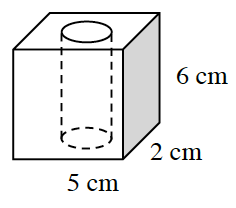### Home > INT2 > Chapter 12 > Lesson 12.2.1 > Problem12-68

12-68.A rectangular prism has a cylindrical hole removed, as shown at right.

1. If the length of the radius of the cylindrical hole is $0.5$ cm, what is the volume of the solid?

Volume of cube − volume of cylinder = volume of solid.

Volume of cylinder $= πr^2h$
$v = π(0.5)^2(6)$
$v = 1.5π ≈ 4.71\text{ cm}^3$

Volume of a cube $= l · w · h$
$v = 5(2)(6) = 60\text{ cm}^3$

Volume of cube − volume of cylinder $= 60\text{ cm}^3-4.71\text{ cm}^3$

$≈ 55.3\text{ cm}^3$

2. What could this geometric figure represent? That is, if it were a model for something that exists in the world, what might it be? Also, how might you change it to make it a better model?